# Difference Between grep() vs. grepl() in R

• Last Updated : 26 Jan, 2022

In this article, we will discuss the difference between grep() and grepl() in R programming language.

## grep()

This grep() function in R Language allows programmers to search for a match of a particular pattern in the given collection of strings. The syntax is given below,

Syntax:  grep(stringPattern, x, ignore.case=TRUE or FALSE, value=TRUE or FALSE)

Parameters:

• stringPattern: A pattern that has to be matched with given elements of the string.
• x: Specified string vector.
• ignore.case: If its value is TRUE, it ignores case.
• value: If its value is TRUE, it return the matching elements vector, else return the indices vector.

Example 1:

## R

 `# R program to illustrate``# grep function`` ` `# Initializing a string vector``x <- ``c``(``"GeeksforGeeks"``, ``"Bhuwanesh"``, ``"Nainwal"``, ``"gfg"``)`` ` `# Calling grep() function``grep``(``"GeeksforGeeks"``, x)``grep``(``"Bhuwanesh"``, x)``grep``(``"gfg"``, x, ignore.case = ``FALSE``)``grep``(``"Nainwal"``, x, ignore.case = ``TRUE``)`

Output: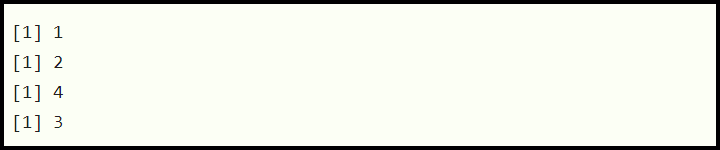Example 2:

## R

 `# R program to illustrate``# grep function`` ` `# Creating string vector``x <- ``c``(``"GeeksforGeeks"``, ``"Bhuwanesh"``, ``"Nainwal"``, ``"gfg"``)`` ` `# Calling grep() function``grep``(``"gfg"``, x, ignore.case = ``TRUE``, value = ``TRUE``)``grep``(``"Bhuwanesh"``, x, ignore.case = ``TRUE``, value = ``TRUE``)``grep``(``"GeeksforGeeks"``, x, ignore.case = ``FALSE``, value = ``FALSE``)``grep``(``"Nainwal"``, x, ignore.case = ``FALSE``, value = ``FALSE``)        `

Output: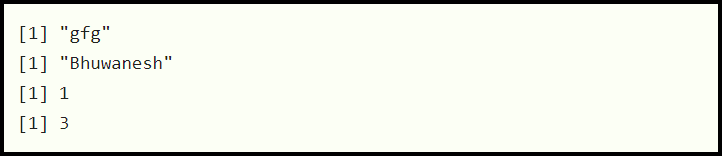## grepl()

This grepl() function in the R language returns the value True if the specified pattern is found in the vector and false if it is not found.

The syntax is given below,

Syntax: grepl(stringPattern, string, ignore.case=FALSE)

Parameters:

• stringPattern: The string pattern to be searched
• string: character vector on which searching to be performed
• ignore.case: whether to ignore case in the search. Here ignore.case is an optional parameter as is set to FALSE by default.

Example 1:

## R

 `# R program to illustrate``# grepl function`` ` `# Initializing a string vector``str <- ``c``(``"GeeksforGeeks"``, ``"Bhuwanesh"``, ``"Nainwal"``, ``"gfg"``)`` ` `# Calling grepl() function``grepl``(``"GeeksforGeeks"``, str)``grepl``(``"Bhuwanesh"``, str)``grepl``(``"gfg"``, str)``grepl``(``"Nainwal"``, str)`

Output: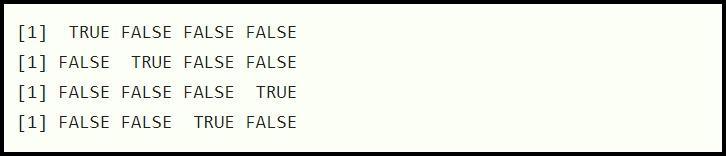Example 2:

## R

 `# R program to illustrate``# grepl function`` ` `# Creating string vector``x <- ``c``(``"GeeksforGeeks"``, ``"Bhuwanesh"``, ``"Nainwal"``, ``"gfg"``)`` ` `# Calling grepl() function``grepl``(``"gfg"``, x, ignore.case = ``TRUE``)``grepl``(``"Bhuwanesh"``, x, ignore.case = ``TRUE``)``grepl``(``"GeeksforGeeks"``, x, ignore.case = ``TRUE``)``grepl``(``"Nainwal"``, x, ignore.case = ``TRUE``)        `

Output:## Difference between grep() and grepl()

Most of the time these two functions are considered the same. Though both the functions are used to check whether a particular pattern matches in the given collection of strings but they differ in the types of output returned by them.

• grep(): This function returns a vector of indices of the character strings that contain the pattern.
• grepl(): This function returns TRUE if a pattern exists in a character string.

Example:

In this example, we are searching the pattern “GeeksforGeeks” in the data vector using grep() function, it returns 1 since this pattern is located at the index 1 in the given vector. Also, we are searching the pattern “Bhuwanesh” in the same vector but using grepl() function this time, and it returns a set of boolean values describing whether the ith element of the vector contains this pattern or not.

## R

 `# create a vector of data``data <- ``c``(``"GeeksforGeeks"``, ``"gfg"``, ``"Bhuwanesh"``,``          ``"Nainwal"``, ``"Swift"``)`` ` `grep``(``"GeeksforGeeks"``, data)``grepl``(``"Bhuwanesh"``, data) `

Output: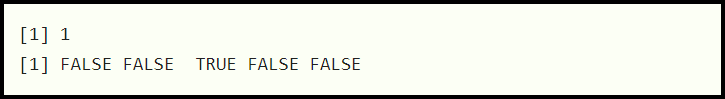## When grep() should be used?

grep is preferred to opt select columns based on the name of the column.

Example: In this example, we have selected entire columns having the character ‘S’ in the header name.

## R

 `library``(dplyr)`` ` `# creating a data frame``df <- ``data.frame``(Department = ``c``(``'CSE'``, ``'IT'``,``                                ``'ECE'``, ``'EE'``, ``                                ``'ME'``),``                 ``Strength = ``c``(80, 76, 75, 65, 70),``                 ``Score = ``c``(75, 70, 65, 60, 60))`` ` `# select columns that contain the string ``# 'S' in their name``df %>% ``select``(``grep``(``'S'``, ``colnames``(df)))`

Output: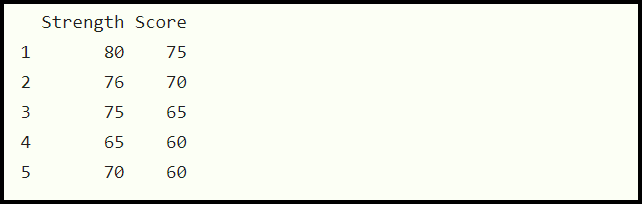Count the Number of Rows that Contain a Certain String. grep() function should be used to count the number of rows in the given data frame that matches with a certain string.

Example: In this example, we have counted the number of rows, in which the header has ‘S’ in it.

## R

 `library``(dplyr)`` ` `# creating a data frame``df <- ``data.frame``(Department = ``c``(``'CSE'``, ``'IT'``, ``'ECE'``,``                                ``'EE'``, ``'ME'``),``                 ``Strength = ``c``(80, 76, 75, 65, 70),``                 ``Score = ``c``(75, 70, 65, 60, 60))`` ` `# select and count columns that contain``# the string 'S' in their name``df %>% ``length``(``grep``(``'S'``, ``colnames``(df)))`

Output: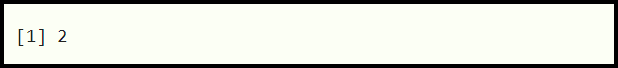## When grepl() should be used?

grepl() should be used for filtering rows in a data frame that contains a particular string.

Example: In this example, we have filtered rows based on the strength value 75.

## R

 `library``(dplyr)`` ` `# creating a data frame``df <- ``data.frame``(Department = ``c``(``'CSE'``, ``'IT'``, ``'ECE'``, ``                                ``'EE'``, ``'ME'``),``                 ``Strength = ``c``(80, 75, 75, 65, 70),``                 ``Score = ``c``(75, 70, 65, 60, 60))`` ` `# filter rows that contain the string``# 75 in the Strength column``df %>% ``filter``(``grepl``(75, Strength))`

Output: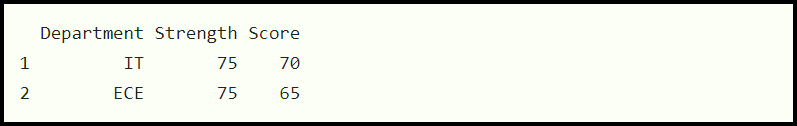My Personal Notes arrow_drop_up